Next: Worked example 3.4: Hail Up: Motion in 3 dimensions Previous: Worked example 3.2: Gallileo's

## Worked example 3.3: Cannon shot

Question: A cannon placed on a 50m high cliff fires a cannonball over the edge of the cliff at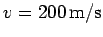making an angle of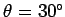to the horizontal. How long is the cannonball in the air? Neglect air resistance.

Answer: In order to answer this question we need only consider the cannonball's vertical motion. At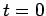(i.e., the time of firing) the cannonball's height off the ground is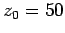m and its velocity component in the vertical direction is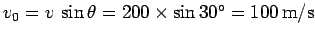. Moreover, the cannonball is accelerating vertically downwards at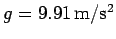. The equation of vertical motion of the cannonball is written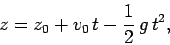whereis the cannonball's height off the ground at time. The time of flight of the cannonball corresponds to the timeat which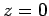. In other words, the time of flight is the solution of the quadratic equation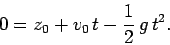Hence,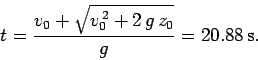Here, we have neglected the unphysical negative root of our quadratic equation.Next: Worked example 3.4: Hail Up: Motion in 3 dimensions Previous: Worked example 3.2: Gallileo's
Richard Fitzpatrick 2006-02-02